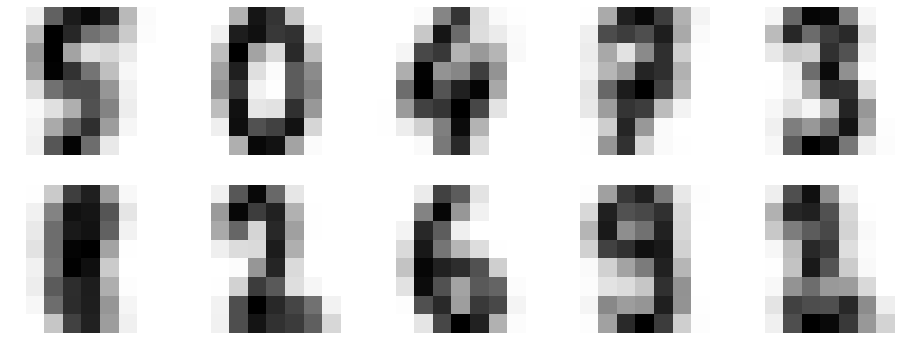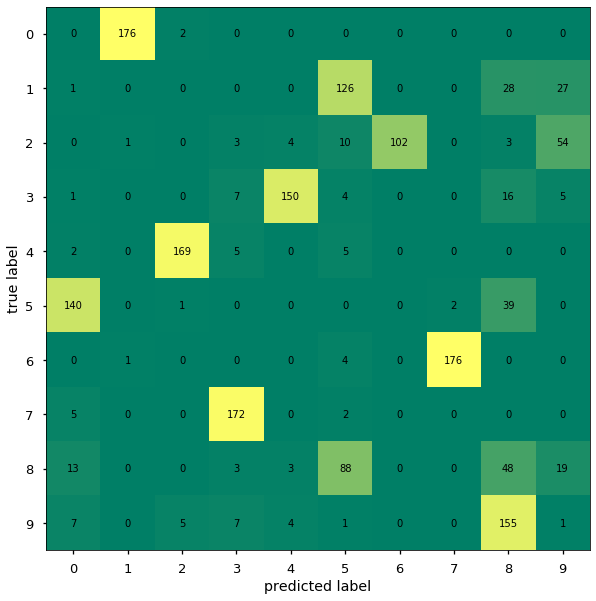# 08.06 Evaluate Clusters¶

One detail we left until about unsupervised learning is the evaluation of the model performance. In general evaluating unsupervised learning is much harder than supervised learning because we cannot simply take the labels and perform cross validation or use a test set. That being said, most evaluations for unsupervised learning do require labels to be known but cross validation or test sets often do not make sense in these evaluations.ul-write.svg

We did see that the dataset of handwritten digits has non-linear patterns, hence it is quite a difficult problem for a technique that is linear. On the other hand, the digits dataset has a very known number of clusters: exactly $10$ clusters, the digits from $0$ to $9$.

Hence we import the dataset loader and MiniBatchKMeans, a k-means implementation that is the de-facto standard for k-means use. Compared to our earlier implementation MiniBatchKMeans is optimized to work with enormous amounts of data, even with more data than the computer memory can hold. We also import the metrics module which we will use to evaluate the performance of our algorithm.

In :
import numpy as np
import matplotlib.pyplot as plt
%matplotlib inline
plt.style.use('seaborn-talk')
from sklearn.cluster import MiniBatchKMeans
from sklearn import metrics


Without knowing of any labels k-means can deal with quite complex problems. Note that we known the labels for the digits dataset and we will indeed use the labels later for the evaluation. But we will not tell k-means about the labels.

In :
digits = load_digits()
digits.data.shape

Out:
(1797, 64)

One of the hyperparameters to MiniBatchKMeans is the number of clusters, which for the digits is easy to know.

Let's see what k-means can take out of that data.

In :
kmeans = MiniBatchKMeans(n_clusters=10)
clusters = kmeans.fit_predict(digits.data)
kmeans.cluster_centers_.shape

Out:
(10, 64)

We used fit_predict, which is similar to fit_transform. We have build the model (the fit part) and predicted the classes based on the cluster centers built by this model. Contrary to supervised learning, it is common to predict on the same data in unsupervised learning. This is because this way we can evaluate the model against other measures on the same data. It is worth to repeat this, in supervised learning predicting (and scoring) the same data that was used for training/fitting is very bad practice; in unsupervised learning it is common and good practice.

The cluster centers are shaped just like the images we worked with. Since we are clustering in the same number of dimensions as the images. And we can build images from the cluster centers.

In :
fig, axes = plt.subplots(2, 5, figsize=(16, 6))
centers = kmeans.cluster_centers_.reshape(10, 8, 8)
for ax, center in zip(axes.flat, centers):
ax.imshow(center, cmap='binary')
ax.axis('off')These are not images of the digits from the dataset, there are cluster centers. Some of them look similar to known digits, others are harder to tell. I will argue that $0$ and $6$ are very visible in this case, we will see whether it translates to the evaluation later. Note that k-means starts from random centers, if you run this code yourself several times you will get different outputs but the cluster centers will look similar enough.

Remember that kmeans was never told what labels these digits, it can only know that it should look for $10$ different digits in the data. Hence, in order in which we plotted the centers, k-means calls these clusters $0$, $1$, $2$, and so on. Whenever the cluster index align with the actual digit in the cluster is out of pure luck.

In :
clusters.shape, np.unique(clusters)

Out:
((1797,), array([0, 1, 2, 3, 4, 5, 6, 7, 8, 9], dtype=int32))

The default k-means scoring is by cluster inertia, the sum of all distances form points to its cluster center. Where the higher the sum is the worse is the clustering. This allows to compare two k-means runs against each other. Notably, sklearn initializes k-means several times and then compares the inertia.

But cluster inertia is not a good measure to compare k-means between datasets or against other models. Cluster inertia and other similar techniques (e.g. within cluster sum of squares) perform certain assumption about cluster shape and evaluate whether the resulting clusters follow that shape. The strongest assumption is that all clusters are round, i.e. have the same shape in every direction/dimension. In sklearn the inertia sum score is negated (made negative) so that the highest value is can achieve is zero, the perfect inertia score. This done by sklearn to such measures that are considered to be worse as they increase, it is made for consistency with positive scores.

Comparing unsupervised algorithms between themselves is always a tricky area. Here we know the labels, therefore we can use better scoring techniques.

In :
(metrics.v_measure_score(digits.target, clusters),

Out:
(0.7341730320210946, 0.7314852717465042)

Both the v-measure and mutual information are scoring techniques that require the knowledge of the labels. Yet, they work differently from F1 or accuracy scores. For example if we have a $3$ class dataset: ships, cars and planes and:

• All cars are clustered together as planes,
• all ships are clustered together as cars,
• and all planes are clustered together as ships.

The we have a perfect ($1.0$) score. In other words the scoring ignores the values of the labels, it only checks whether the labels are together in the same cluster.

For an algorithm that only knew that there are $10$ digits in the data but had no idea how they look like that is a pretty amazing score. Let's look at what got misclassified the most.

A classification report would not give us a good visualization because it assumes that the same labels are classified as themselves. Instead we will build a confusion matrix, which gives us what labels were assigned to each set of other labels. We can then evaluate which classes the model is better at identifying, even without knowing which class should be identified as what.

Note that we cannot give accurate ticks (labels) to the predicted values, we can only guess their value from the ticks on the data we know. The confusion_matrix procedure produces the matrix with the true labels, first arguments, as rows and the predicted labels, second argument, as the columns of the matrix. This order of arguments and matrix order is important to remember, it is easy to confuse oneself as to which are the true and which the predicted labels and end with a transposed matrix. That said, this order of matrix presentation is specific to sklearn, a confusion matrix produced by other libraries may produce a transposed version of the matrix.

In :
from sklearn.metrics import confusion_matrix

mat = confusion_matrix(digits.target, clusters)
fig, ax = plt.subplots(figsize=(10, 10))
cax = ax.matshow(mat, cmap='summer')
ticks = np.arange(0,10,1)
ax.set_xticks(ticks)
ax.set_yticks(ticks)
ax.set_xticklabels(ticks)
ax.set_yticklabels(ticks)
ax.set_ylabel('true label')
ax.set_xlabel('predicted label')
ax.xaxis.set_ticks_position('bottom')

for i in range(mat.shape):
for j in range(mat.shape):
ax.text(j, i, mat[i, j], ha='center', va='center')
mat

Out:
array([[  0, 176,   2,   0,   0,   0,   0,   0,   0,   0],
[  1,   0,   0,   0,   0, 126,   0,   0,  28,  27],
[  0,   1,   0,   3,   4,  10, 102,   0,   3,  54],
[  1,   0,   0,   7, 150,   4,   0,   0,  16,   5],
[  2,   0, 169,   5,   0,   5,   0,   0,   0,   0],
[140,   0,   1,   0,   0,   0,   0,   2,  39,   0],
[  0,   1,   0,   0,   0,   4,   0, 176,   0,   0],
[  5,   0,   0, 172,   0,   2,   0,   0,   0,   0],
[ 13,   0,   0,   3,   3,  88,   0,   0,  48,  19],
[  7,   0,   5,   7,   4,   1,   0,   0, 155,   1]])The matshow procedure works in the same way as imshow, give a matrix it colors the values a pixels (or squares). Note that showing images, or matrices in this case, reverses the vertical axis. The numbers on the vertical increase as they go downwards, this is contrary to normal plots. And thanks to this reversing of the vertical axis we needed to force the tick position (set_ticks_position) on the horizontal axis.

As we noted before, every k-means run will produce a different matrix but the general patterns will be similar. In the specific case here we can argue that (first row) almost all zeros were assigned to the same cluster - cluster $1$. The ones are rather varied but most of them have been assigned to cluster $5$. And a similar analysis can be made for every row.

Earlier we said that we have very good cluster centers for $0$ and $6$. And this is indeed true row $0$ and row $6$ - assigned to clusters $1$ and $7$ are almost all assigned to the same cluster each.

Often a confusion matrix is seen in the context of supervised learning, and eventually we will see one in this context. In supervised learning one argues that the main diagonal, form the top left to the bottom right should hold the biggest values. Here in unsupervised learning we do not know what the labels on the horizontal axis should be, hence that argument is not appropriate.

Since we can assign the cluster centers any names we like we can move the columns of this matrix freely, and doing that we could achieve the majority of the big numbers in the main diagonal. But that is beyond the point of the confusion matrix, we use the matrix to check whether the clusters pick mostly the same digit. In other words, we are checking whether we have one big number per row and then just a few numbers that are not zero. A perfect clustering evaluation would have just a single number per column and per row. Whether such a perfect classification would work on new, previously unseen data, is a hard problem. But one can reasonably say that a good enough clustering would produce good enough generalization results.

Now, given new data we can ask our k-means model in which cluster it lives. The model will tell us the label of the cluster, we then can look into the confusion matrix and say what that label maps to according to the true labels. For example, given a new image to which our k-means predicts $9$ we can be rather confident that the image is of a $7$. This may be a lot of trouble to go through for a prediction that could be made directly with supervised learning but one must remember that k-means would still work if we had no labels at all or just a small handful of labels in our dataset. Contrary to a supervised learning algorithm.